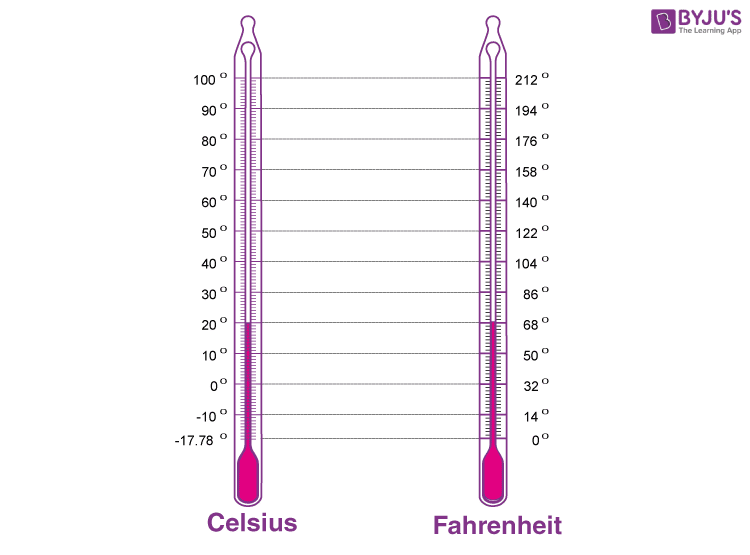Checkout JEE MAINS 2022 Question Paper Analysis : Checkout JEE MAINS 2022 Question Paper Analysis :

# Difference Between Celsius And Fahrenheit

We know that temperature is the measure of the degree of hotness or coldness. Temperature is a physical quantity that is measurable. Celsius and Fahrenheit are the commonly used units to measure temperature. In this article, learn the difference between Celsius and Fahrenheit.

## Units of Temperature

There are many units to measure temperature, but the most popular unit to measure the temperature in Celsius and Fahrenheit. These two units have respective values for boiling and freezing points, with reference to water. Celsius and Fahrenheit, the temperature measuring units are very useful in many ways and are used all over the world. There are formulas to convert from Celsius to Fahrenheit, click here for Celsius to Fahrenheit Conversion. The difference between Fahrenheit and Celsius is given below to help learners understand the concept more effectively.### Celsius

Celsius is also called centigrade and is used by most countries. The Celsius is named from a Swedish Astronomer called Andres Celsius, who introduced a similar instrument to measure the temperature.

### Fahrenheit

Fahrenheit system is used in the United States for measuring temperature as a standard for non-scientific uses. It is named after a German-Dutch Physicist named Daniel Gabriel Fahrenheit.

## Temperature Differences Between Celsius And Fahrenheit

 Temperature Differences Between Celsius And Fahrenheit Celsius Fahrenheit Absolute Zero -273.15 -459.67 Boiling Point of Water 99.9839 211.97102 Melting Point of Ice 0 32 Average Human Body Temperature 37.0 98.6

Hope you have understood the differences between Celsius And Fahrenheit. Stay tuned with BYJU’S to learn more about temperature, the impact of temperature, and much more.
See the video below, to know what is temperature.## Frequently Asked Questions – FAQs

### What is temperature?

Temperature is the measure of the degree of hotness or coldness.

### What are the unit to measure the temperature?

Celsius and Fahrenheit are the units to measure the temperature.

### What is the melting point of ice in Celsius?

The melting point of ice is zero degrees Celsius.

### What is the average human body temperature in Fahrenheit?

The average human body temperature is 98.6 Fahrenheit.

### Who invented the Fahrenheit system?

The Fahrenheit system was introduced by Daniel Gabriel Fahrenheit.

Test your knowledge on Celsius and Fahrenheit Difference